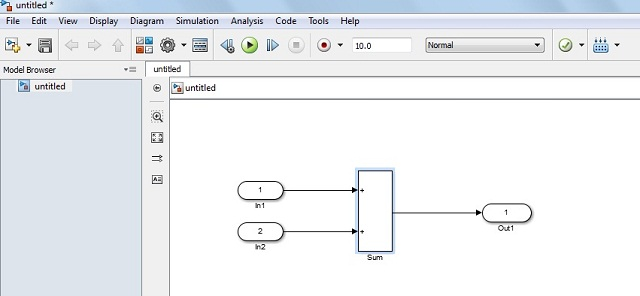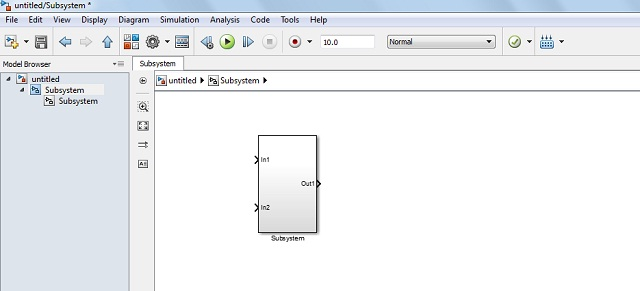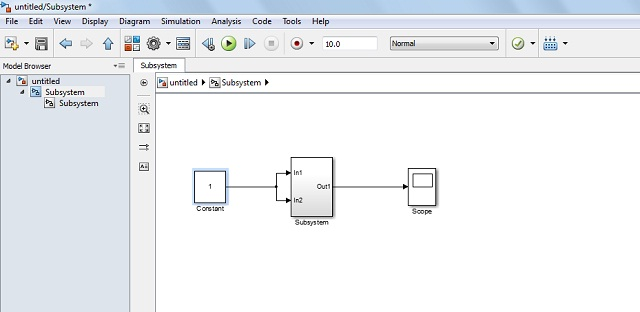# How to Create MATLAB Simulink Subsystem Model? [Explained with Example]

Today I am describing and demonstrating- ‘How to make a Subsystem model in MATLAB Simulink?’

When I tried to make the subsystem model in the Simulation model the first time, I felt, it is more critical. After that, I have recognized, it is not much difficult to make the subsystem.

Let’s see how.

The purpose of this tutorial is to learn and understand it easily.### What is Subsystem Model?

In MATLAB Simulink, various types of the block are used in the model as per our requirement. And these blocks are connected to each other. As we start developing the project, these model size gets increased and so the complexity.

Here, we have a better solution by creating the subsystem model.

The subsystem is created by grouping the multiple blocks.

So we can define it as…

The various number of blocks are converted into a single block then this single block is called a Subsystem model.

### Creating a MATLAB Simulink Subsystem Model

We can distinguish this operation into three steps…

• Converting Given Model into Subsystem Model
• Connecting Blocks to the Subsystem Model

Let’s see each step one-by-one.

To make this simple, in this tutorial we are going to create a Subsystem model for Addition.

• Open MATLAB software. If you are a new MATLAB user, you can refer tutorial to start and run the first Simulink model.
• First of all, click on the File menu and then click on New Model for creating a new model.
• It will open Simulink libraries.
•  Choose Math Operations, Source and Sink components to create your first model for the addition operation.

Now, the new model consists of a single sum block, source as two input and sink as a single output. These blocks are connected with each other by the straight line as shown in the image shown below.#### Step II: Creating a Subsystem model

It is very easy. Follow the steps given below.

• Select the complete addition model. And then click on the topmost menu option Diagram.
• Choose the Subsystem and Reference model menu option from the Diagram.

This will convert the addition model into the form of the subsystem block. It will look like a single block as shown in the image below.#### Step III: Connecting Subsystem Model to Other Blocks

• After creating the subsystem model, connect the input and output to the other blocks as per shown in the image below.The Subsystem Model with Connected Input and Output Block

Note: You can connect any blocks to the Subsystem as per your project requirement.

You can see the internal blocks of the subsystem model by double-clicking on it.

### Advantage Of Creating Subsystem Model

Rather than making a single model from all the required blocks, Subsystem model has many advantages of creating and adding a subsystem model.

Why should you create a Subsystem model in Simulink?

• Subsystem model is very easy to read out and to understand.
• It helps to reduce the number of blocks displayed in your actual model.
• It reduces the complexity of the simulation model.
• Inside the Subsystem model, you can keep functionally related blocks together.

This is all about creating your first MATLAB Simulink subsystem model. If you have any questions or difficulty creating subsystem model, feel free to ask me in the comment section below.

Will see you soon with the next MATLAB tutorial. Till then, bye!

Practice Now »

### 16 thoughts on “How to Create MATLAB Simulink Subsystem Model? [Explained with Example]”

1.So..Nice..

•Thank you so much!

2.Hello, I have checked your article. It is a good and simple explanation.

•Thank you, Richard.

3.Nice tutorial, Thank you

4.Nice article.

•Thanks, Ketki 🙂

5.Good, explanation!

•Thanks, Priya:)

6.•Thanks, Ramand 🙂

7.It is a very informative article.

•Thanks, Smith 🙂

8.what a article mam

9.•